5G - Massive MIMO                                                          Home : www.sharetechnote.com Massive MIMO Channel Model - Examples   Following is based on Multi-Layer Precoding for Full-Dimensional Massive MIMO Systems (  ).   This paper is based on the situation as illustrated below. I extended the illustration from the paper to make it more closely match the mathematical expression in the paper.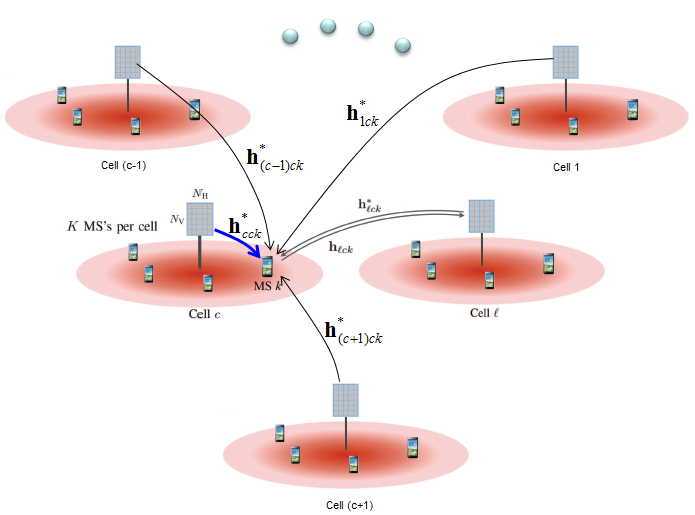As in any other channel model, this paper starts with system model. The system model for this environment is described as follows.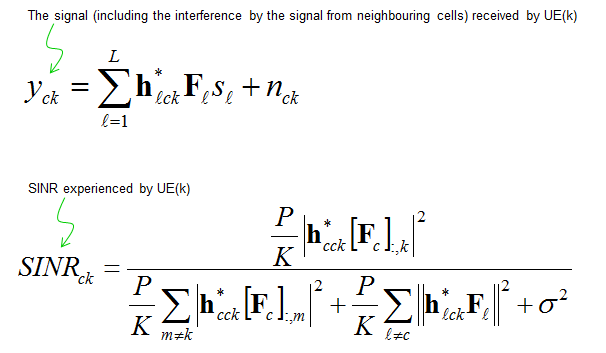The main purpose of this paper is to design (derive) precoding matrix as labeled below.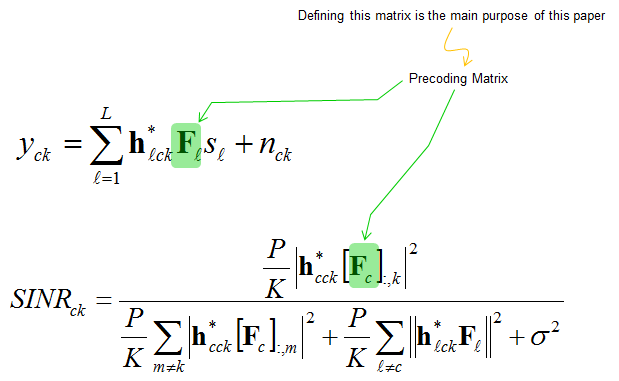Describing the received signal model, it is as follows.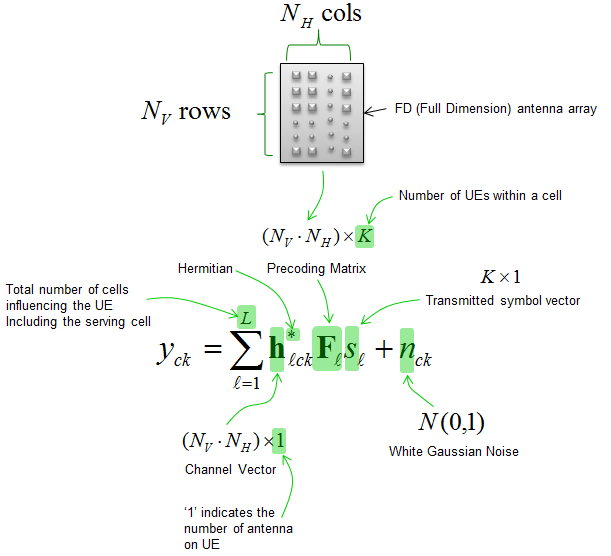If you look into the details of SINR model, it can be commented as follows.Now the questions is how to define (derive) the precoding matrix F. This paper defines three separate matrix each of which is specifialized for only one purpose as described below.  I just put down the big picture here, if you are interested in the details of this matrix, refer to the original paper (Ref )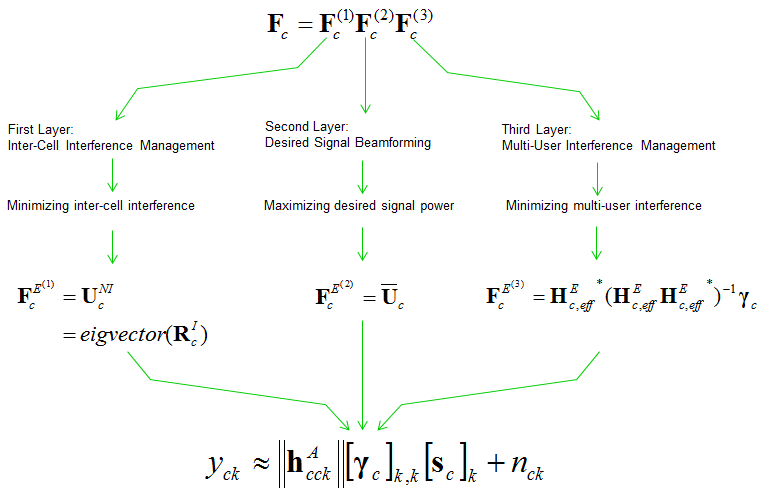Reference    Multi-Layer Precoding for Full-Dimensional Massive MIMO Systems Ahmed Alkhateeby, Geert Leusz, and Robert W. Heath Jr.y The University of Texas at Austin, TX, USA, Email: faalkhateeb, rheathg@utexas.edu Delft University of Technology, The Netherlands, Email: g.j.t.leus@tudelft.nl